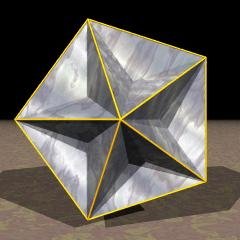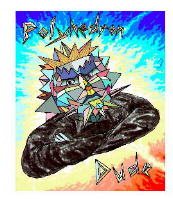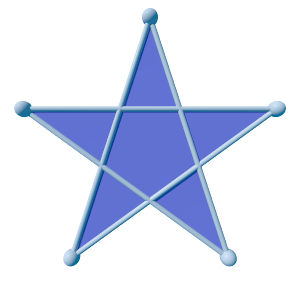TOCID symbol E
Name great dodecahedron,
faceted icosahedron,
vertex figure of fix

`© ©`
Density 3
Vertex figure /2
Vertex layers
 Layer Symmetry Subsymmetries o5o5/2o o5o   . o .   o . o5/2o 1 x5o5/2o o5o   . x .   oedge first . o5/2overtex first 2 x5o   .{5} first o .   f . f5/2overtex figure 3 o5x   .opposite {5} f .   x . o5/2f 4 o5o   . o .   f . o5/2oopposite vertex 5 x .   oopposite edge
Lace city
in approx. ASCII-art
``` o   o
f
x     x
f
o   o
```
Coordinates (τ/2, 1/2, 0)   & even permutations, all changes of sign
where τ = (1+sqrt(5))/2
General of army ike
Colonel of regiment ike
Dual sissid
Dihedral angles
• between {5} and {5}:   arccos(1/sqrt(5)) = 63.434949°
Confer
Grünbaumian relatives:
compounds:
presipsido   presipsi
general polytopal classes:
regular   noble polytopes
ExternalAs abstract polytope gad is isomorphic to sissid, thereby replacing facial pentagons by pentagrams and conversely vertex figure pentagrams by corresponding pentagons. – As such gad is a lieutenant. Both gad and sissid can be seen as different realizations of the same self-dual regular abstract polyhedron {5,5}6 (where the index just denotes the size of the corresponding Petrie polygon).

This polyhedron is an edge-faceting of the icosahedron (ike).

Incidence matrix according to Dynkin symbol

```o5/2o5x

.   . . | 12 |  5 |  5
--------+----+----+---
.   . x |  2 | 30 |  2
--------+----+----+---
.   o5x |  5 |  5 | 12

snubbed forms: o5/2o5β
```

```o5/3o5x

.   . . | 12 |  5 |  5
--------+----+----+---
.   . x |  2 | 30 |  2
--------+----+----+---
.   o5x |  5 |  5 | 12
```

```x5/4o5/2o

.   .   . | 12 |  5 |  5
----------+----+----+---
x   .   . |  2 | 30 |  2
----------+----+----+---
x5/4o   . |  5 |  5 | 12
```

```x5/4o5/3o

.   .   . | 12 |  5 |  5
----------+----+----+---
x   .   . |  2 | 30 |  2
----------+----+----+---
x5/4o   . |  5 |  5 | 12
```